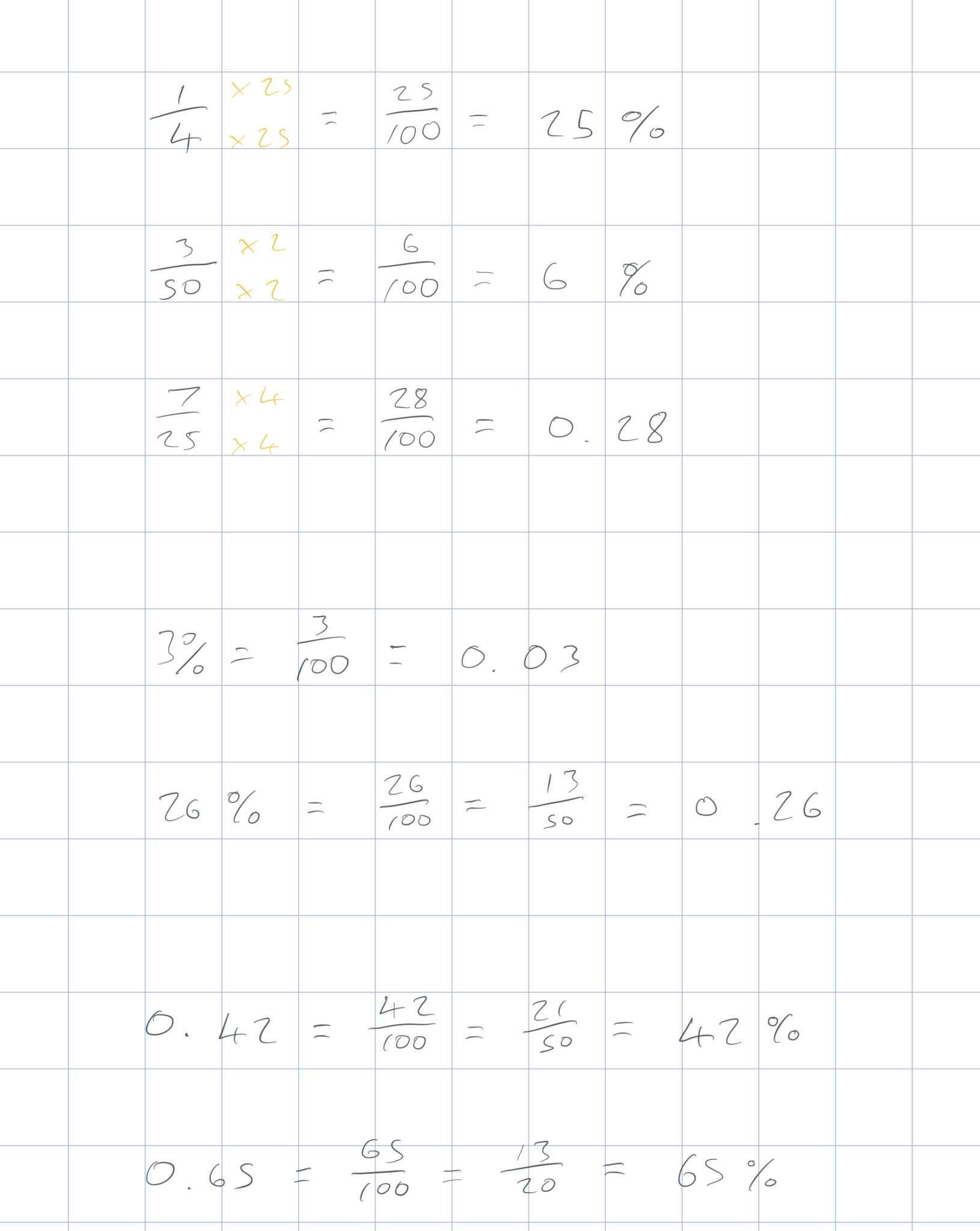To convert from a fraction to a percentage, make the denominator of the fraction 100 by multiplying or dividing the top and bottom of the fraction by the same number.

To convert from a fraction to a decimal, make the denominator 10, 100 or 1000 by multiplying or dividing the top and bottom of the fraction by the same number and use knowledge of place value. e.g. 3/10 = 0.3, 17/100 = 0.17, etc.

To convert from a percentage to a decimal, write as a fraction and use knowledge of place value.

To convert from a decimal to a fraction, use knowledge of place value to write as a fraction over 10, 100 or 1000, then simplify, e.g. 0.12 = 12/100 = 6/50 = 3/25.

To convert from a decimal to a percentage, write the decimal as a fraction over 100 then write as a percentage, e.g. 0.47 = 47/100 = 47%.